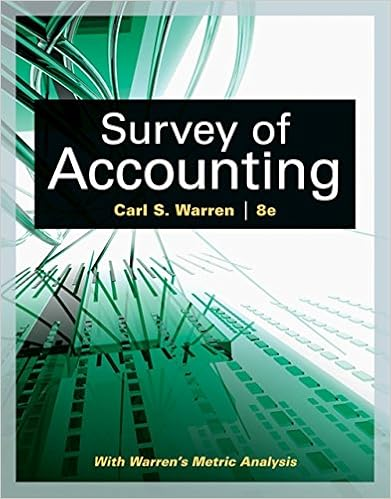# Alternatively offer an investor a choice between two

• Notes
• 326
• 100% (5) 5 out of 5 people found this document helpful

This preview shows page 188 - 191 out of 326 pages.

##### We have textbook solutions for you!
The document you are viewing contains questions related to this textbook.The document you are viewing contains questions related to this textbook.
Chapter 6 / Exercise MBA 6-1
Survey of Accounting
WarrenExpert Verified
Alternatively, offer an investor a choice between two investments, and change the possible returns (or probabilities) until the investor is indifferent between the two investments. Suppose the investor is indifference between investments A and B then U ( E ( r A ) , σ A ) = U ( E ( r B ) , σ B ) (7.2) E ( r A ) - 0 . 5 2 A = E ( r B ) - 0 . 5 2 B (7.3) Solve the resulting equation for A . Example: Suppose an investor is indifferent between the following two investment alternatives: 15% 5% 8% -5% p = 0.40 p = 1.00 To be able to measure risk by σ (i.e., in the mean-variance framework), the probability distribution of the risky investment must be normal. This example uses a discrete distribution only to show the calculation process. For the first investment, E ( r ) = 5% and σ = 0%, and therefore U = 5% - 0 . 5 × A × 0% 2 = 5% For the second investment, E ( r ) = 0 . 30 × 15% + 0 . 40 × 8% + 0 . 30 × - 5% = 6 . 20% σ = p 0 . 30(15% - 6 . 20%) 2 + 0 . 40(8% - 6 . 20%) 2 + 0 . 30( - 5% - 6 . 20%) 2 = 7 . 88% U = 6 . 20% - 0 . 5 × A × 7 . 88% 2 From indifference: 5% = 6 . 20% - 0 . 5 × A × 7 . 88% 2 A = 6 . 20% - 5% 0 . 5 × 7 . 88% 2 = 3 . 861003861 3 . 86
##### We have textbook solutions for you!
The document you are viewing contains questions related to this textbook.The document you are viewing contains questions related to this textbook.
Chapter 6 / Exercise MBA 6-1
Survey of Accounting
WarrenExpert Verified
Risk Aversion and Asset Allocation 7-7 7.6.1 Exercise An investor is indifferent between two investments with the following statistics: E ( r 1 ) = 12 . 00% , σ 1 = 36 . 00% , E ( r 2 ) = 14 . 00% , σ 2 = 37 . 40%. The probability distribution of returns on both the investments is normal. What is the risk aversion parameter ( A ) of this investor? Solution Since the investor is indifferent between the two investments, her utility from the two investments must be equal: U ( E ( r 1 ) , σ 1 ) = U ( E ( r 2 ) , σ 2 ) E ( r 1 ) - 0 . 5 2 1 = E ( r 2 ) - 0 . 5 2 2 = A = E ( r 2 ) - E ( r 1 ) 0 . 5 × ( σ 2 2 - σ 2 1 ) = 0 . 14 - 0 . 12 0 . 5 × (0 . 374 2 - 0 . 36 2 ) = 0 . 02 0 . 0051 = 3 . 8925652 3 . 89 7.7 Preference and Dominance If all risk-averse investors, regardless of their degree of risk aversion, prefer one investment over another, the former is said to dominate the latter. If E ( r 1 ) > E ( r 2 ) and σ 1 σ 2 , investment 1 dominates investment 2 since it offers a higher expected return for the same or lower amount of risk. All risk-averse investors will pick 1 over 2. If σ 1 < σ 2 and E ( r 1 ) E ( r 2 ), investment 1 dominates investment 2 since it offers a lower risk for the same or higher amount of expected return. All risk-averse investors will pick 1 over 2. Example: E ( r 1 ) = 14%, σ 1 = 30%, E ( r 2 ) = 16%, σ 2 = 30%. 2 dominates 1. A B C D E ( r ) Dominance and Preference
7-8 Investment Analysis (FIN 756) Course Guide - Fall 2018 C will be preferred over A by all risk-averse investors because C offers a higher expected return for the same risk as A. So, C dominates A. For a similar reason, D dominates B. C dominates D since C offers the same expected return for a lower risk. Likewise, A dominates B. C dominates B because C offers a higher expected return for less risk than B. There is no dominance between A and D. D has a higher expected return and a higher risk. Some investors will be indifferent between A and D. Investors with lower risk aversion will prefer D.
•••